# Expression plus minus

Evaluate expression: (-1)2 . 12 – 6 : 3 + (-3) . (-2) + 22 – (-3) . 2

x =  8

### Step-by-step explanation: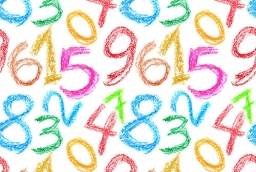Did you find an error or inaccuracy? Feel free to write us. Thank you!## Related math problems and questions:

• Evaluate - order of opsEvaluate the expression: 32+2[5×(24-6)]-48÷24 Pay attention to the order of operation including integers
• Evaluate expressionIf x=2, y=-5 and z=3 what is the value of x-2y
• Expression 6Evaluate expression: -6-2(4-8)-9
• With bracket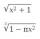Calculate (evaluate) simple mathematical expression with a negative numbers and a bracket: 13+15*5-2*(-6)
• Evaluate expressionEvaluate expression using BODMAS rule: 1 1/4+1 1/5÷3/5-5/8
• Evaluate expression 2Evaluate expression with negatives: (-3)+4+(-8)+(-6)+4+(-1)
• Expression 8Evaluate this expressions: a) 5[3 + 4(2.8 - 3)] b) 5×(8-4)÷4-2
• Value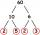Determine the value of the following exspressions: a) (23-25)·(4-5) b) (97-123):(18+8)
• Evaluate 11Multiply the quotient of 6 and 2 by 3, then add 1. Add 1 and 6, then divide by 2 and multiply by 3. Divide 6 by the product of 2 and 3, then add 1.
• DozenWhat is the product of 26 and 5? Write the answer in an Arabic numeral. Add up the digits. How many of this is in a dozen? Divide #114 by this
• Evaluate expr with 2 varsIf a=6 and x=2, find the value of (2ax + 7x- 10) /(4ax-3a-2)
• Evaluate 5Evaluate expression x2−7x+12x−4 when x=−1
• NumberCalculate the integer number which, divided by 34 gives 10 and the rest 25.
• Chocolate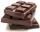How many times do we have to break a chocolate bar composed of 10 × 12 pieces to get the 120 parts?
• Fractions and mixed numerals(a) Convert the following mixed numbers to improper fractions. i. 3 5/8 ii. 7 7/6 (b) Convert the following improper fraction to mixed number. i. 13/4 ii. 78/5 (c) Simplify these fractions to their lowest terms. i. 36/42 ii. 27/45 2. evaluate following ex
• Opposite numbers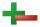Calculate opposite numbers (additive inverse) to given ones:
• MistakeNicol mistake when calculate in school. Instead of add number 20 subtract it. What is the difference between the result and the right result?